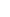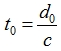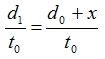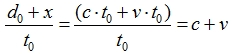6. OUTGOING SIGNAL SPEEDAt this stage, we are going to analyze the speed of the signal that is emitted, namely OUTGOING signal, relative to the reference system sending the signal. We are going to investigate the event for two cases: when the frames are moving away from each other and the frames are approaching each other.6.1. (C+V) STATE, FRAMES ARE MOVING AWAY FROM EACH OTHERWe analyze the situation from the point of Frame A. Frame A thinks that it stands still and that Frame B is moving away from it at v speed.The course of the event relative to Frame As reference system:
1.1  Frame A sends a signal in the direction of Frame B when in d0 distance.
1.2  The signal reaches Frame B at d1 distance.We calculated the travel time of the signal before in the part about INCOMING signals.The travel time of the signal is :Now let's find out the distance:

At the moment the signal sets out, Frame B is at d0 distance to Frame A.
Within the time when the signal sets out and the arrival time, Frame B will travel x distance in the direction of the red arrow.A signal sent from Frame A needs to cover d1 distance in order to reach Frame B.Now that we have information on the distance and the time, we can find the signal speed.Signal speed = distance / time =Therefore, relative to Frame As reference system;OUTGOING signal speed =While the frames are moving away from each other, we can analyze the situation from the point of Frame B by assuming that Frame B is still and Frame A is in motion. However, as we will go through calculations in the exact same way and find the exact same answers, I did not feel the need to write them down here.6.2. (C-V) STATE, FRAMES ARE APPROACHING EACH OTHERWe are going to examine the situation from the point of Frame A. Frame A thinks that it stands still and that Frame B approaches it at v speed.The course of the event relative to Frame As reference system:
1.1  Frame A sends a signal in the direction of Frame B when in d0 distance.
1.2  The signal reaches Frame B at d1 distance.We calculated the travel time of the signal before in the part about INCOMING signals.The travel time of the signal:Now lets find out the distance:
At the moment the signal sets out, Frame B is at d0 distance to Frame A.
Within the time when the signal sets out and arrives, Frame B will travel x distance in the direction of the red arrow.A signal sent from Frame A needs to cover d1 distance in order to reach Frame B.Now that we have information on the distance and the time, we can find the signal speed.Signal speed = distance / time =Therefore, relative to Frame As reference system;OUTGOING signal speed =While the frames are approaching each other, we can examine the situation from the point of Frame B by assuming that Frame B is still and Frame A is in motion. However, since we will go through the calculations in the exact same way and find the exact same answers, I did not feel the need to write them down here.

In the light of the information we got while investigating OUTGOING signals, we can again write a second extremely important invaluable golden result.OUTGOING SIGNALSThe speed of the signal relative to the reference system of the source that emits the signal (electromagnetic wave) is (c+v) if the target of the signal is moving away and (c-v) if the target is approaching. Here c is the speed of light constant and v is the approaching/moving away speed of two reference systems relative to each other.As can be seen, relative to the reference system of the source that emits the signal, in order for the speed that it sends to be c, that is, constant, the arrival target of the signal must be still relative to it. In all situations except this one, the speed of the signal is different from the speed of light.6.3. INCOMING AND OUTGOING SIGNALSIn this way, we have seen that, for a reference system, INCOMING signals that reach and OUTGOING signals that leave the reference system should be handled separately. The missing part here is the fact that OUTGOING signals have not been measured until now. The biggest mistake in physics is right here. While the speed of an OUTGOING signal going towards a moving target should be (c±v), a wrong decision was made as there was no measurement and it was assumed to be c. A relevant measurement will absolutely verify Alice Law and I am telling you this with a clear conscience.Id like to direct your attention to the fact that, while using c value as a base for INCOMING signal speeds, it was founded entirely upon Albert Einsteins Speed of Light Principle. You may think this principle conflicts with OUTGOING signal speeds, but as I said, it is not that simple. What actually matters is the perspective that we adapt while looking at his principle. When you read the last chapter, you may think about this again. I must say that it is impossible to come up with (c+v) (c-v) mathematics without depending on his principle. As you can see here, in order to reach (c+v) (c-v) mathematics, I first used his principle as a base for INCOMING signal speeds and then, again with its help, I found OUTGOING signal speeds by using the time I got (t0 = d0 / c).In this chapter where we obtain (c+v) (c-v) mathematics, you may think that what is written here is so simple and why scientists living in the past couldnt see such a simple thing. Such a judgment would be extremely unfair to all those important people. What I wrote here is not simple at all. Alice Law reached this level of simplicity and clarity slowly and surely and only after so many years. Physics is hard, very hard indeed.6.4. THE ELECTROMAGNETIC THEORY AND (C+V) (C-V) MATHEMATICSI have, in this way, showed you that light and all electromagnetic waves in general behave in accordance with (c+v) (c-v) mathematics. From this point on, I am going to tell you about the results of (c+v) (c-v) mathematics. Now that we have the mathematical foundations and saw how (c+v) (c-v) mathematics was formed, we can easily examine, predict, and comprehend its results and reach many conclusions.Of course, there should be a reason with utmost importance in the nature that led to (c+v) (c-v) mathematics. For now, we are not going to question that reason because the fact that we do not know the reason doesnt change the existence of (c+v) (c-v) mathematics. Therefore, I have decided that it would be more suitable if I told you about topics that I think are more urgent and important. I touched upon the reasons leading to (c+v) (c-v) mathematics in the third and fourth chapter of the book.The fact that I have given my work the name Alice Law and that (c+v) (c-v) mathematics has been included in physics do not change the general situation. The general situation is that all this change is actually formed on The Electromagnetic Theory. We can group this change under three main topics:

• From now on, (c+v) (c-v) mathematics will represent the mathematics of The Electromagnetic Theory. In this way, The Electromagnetic Theory will transform into a form that can correctly express the electromagnetic interaction between reference systems that are moving relative to each other

• The Theory of Relativity will fall off the agenda completely with all its results.

• A solid bridge between The Electromagnetic Theory and Classical Mechanics has been formed.

The biggest responsibility that falls upon the shoulders of scientists at this stage is performing the measurements that they missed and havent done so far. If they measure the speed of a signal going to a moving target, they will empirically confirm the existence of (c+v) (c-v) mathematics.